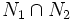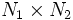# Internal direct product

This article describes a product notion for groups. See other related product notions for groups.

VIEW RELATED: Analogues of this | Variations of this | Opposites of this |[SHOW MORE]

## Definition

### Definition with symbols (for two subgroups)

A group$G$ is termed the internal direct product of two subgroups$N_1$ and$N_2$ if both the following conditions are satisfied:

•$N_1$ and$N_2$ are both normal subgroups
•$N_1$ and$N_2$ are permutable complements, that is,$N_1 \cap N_2$ is trivial and the product of subgroups$N_1N_2 = G$.

Equivalently$G$ is the internal direct product of$N_1$ and$N_2$ if both the following conditions are satisfied:

• Every element of$N_1$ commutes with every element of$N_2$. In other words,$N_1$ is contained in the centralizer of$N_2$.
•$N_1$ and$N_2$ are lattice complements, that is, they intersect trivially and together they generate$G$, i.e., the join of subgroups$\langle N_1,N_2 \rangle$ is equal to$G$.

The two subgroups$N_1$ and$N_2$ are termed direct factors of$G$.

### Definition with symbols (for arbitrary family of subgroups)

A group$G$ is termed the internal direct product of subgroups$N_i, i \in I$, if the following three conditions are satisfied:

1. Each$N_i$ is a normal subgroup of$G$
2. The$N_i$s generate$G$
3. Each$N_i$ intersects trivially the subgroup generated by the other$N_j$s. Equivalently, if$g_1g_r\dots g_r = e$ where$g_l \in N_{j_l}$ with all$j_l$ distinct, then each$g_l = e$.

Equivalently,$G$ is the internal direct product of the$N_i$s if the following two conditions are satisfied:

1. Every element of$N_i$ commutes with every element of$N_j$ for$i \ne j$
2. Each$N_i$ is a lattice complement to the subgroup generated by the remaining$N_j$s

### Equivalence of definitions

Further information: equivalence of definitions of internal direct product

### Equivalence with the external direct product

Further information: equivalence of internal and external direct product

It can be proved that if$G$ is an internal direct product of subgroups$N_1$ and$N_2$, then$G$ is isomorphic to the external direct product$N_1$ ×$N_2$ via the isomorphism that sends a pair$(a,b)$ from$N_1 \times N_2$ to the product$ab$ in$G$. Conversely, given an external direct product$N_1 \times N_2$, we can find subgroups isomorphic to$N_1$ and$N_2$ in the external direct product such that it is the internal direct product of those subgroups.

For infinite collections of subgroups, the internal direct product does not coincide with the external direct product -- instead, it coincides with the notion of restricted external direct product.